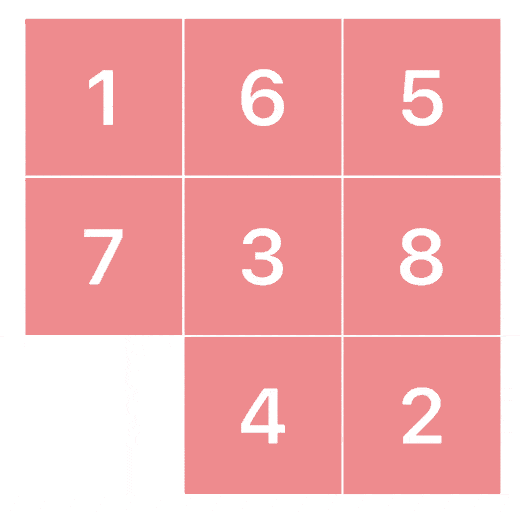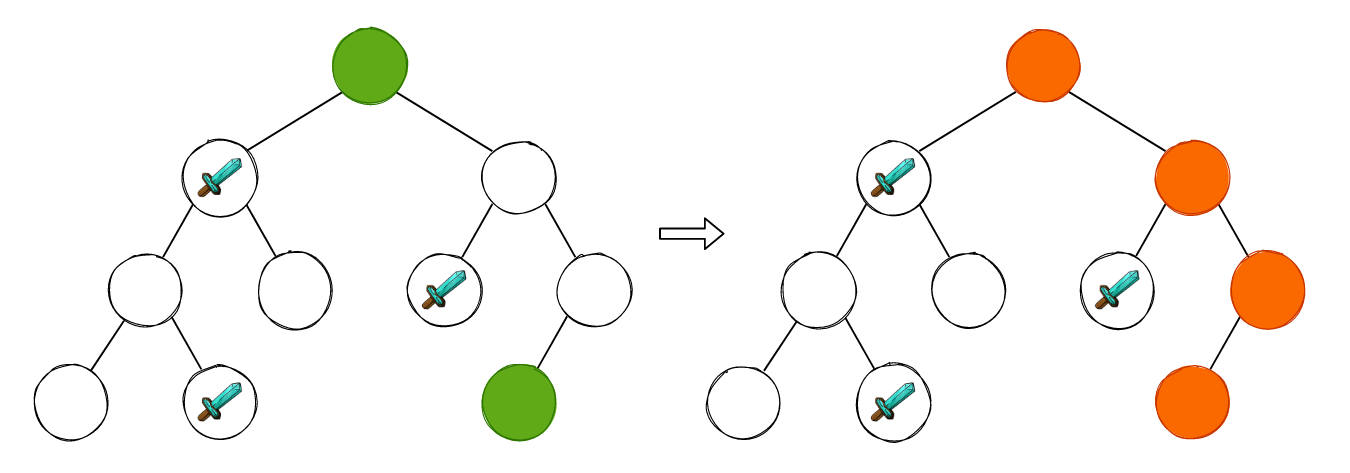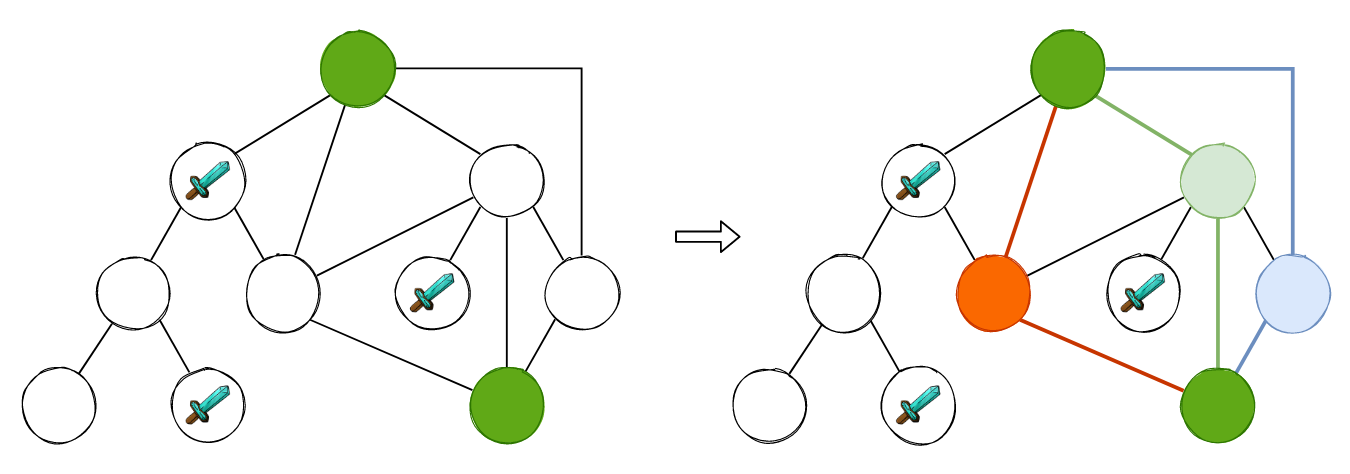### 1. BFS 的基本框架

``````void bfs(int src, int dst) {
vector<bool> visited;
queue<int> levelQueue;

visited[src] = true;
levelQueue.push(src);

while(!levelQueue.empty()) {
int size = levelQueue.size();

// 非常实用的小技巧，取出每一层的数据
for (int i = 0; i < size; i++) {
int current = levelQueue.front();
levelQueue.pop();

for (int neighbor: neighbors(current)) {
if (visited[neighbor]) continue;
visited[neighbor] = true;
// do something for child
levelQueue.push(child);
}
}
}
}
``````

### 2. 直白的 BFS 问题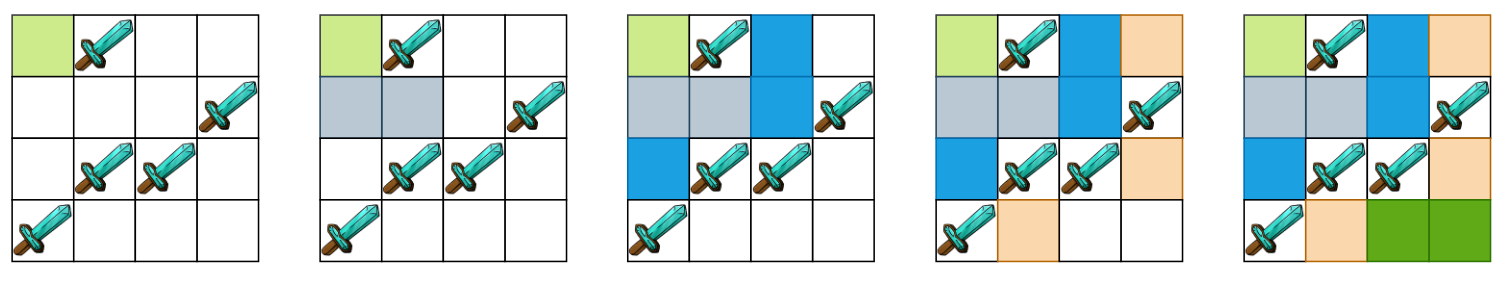``````class Solution {
private:
int m, n;
vector<vector<int>> delta =  {{-1, 0}, {-1, 1}, {0, 1}, {1, 1}, {1, 0}, {1, -1}, {0, -1}, {-1, -1}};
bool inGrid(int x, int y) {
return x >= 0 && x < m && y >= 0 && y < n;
}
public:
int shortestPathBinaryMatrix(vector<vector<int>>& grid) {
m = grid.size(), n = grid.size();

if (grid == 1 || grid[m-1][n-1] == 1) return -1;
if (m == 1 && n == 1) return 1;

queue<pair<int, int>> levelQueue;
vector<vector<int>> visited(m, vector<int>(n, -1));

levelQueue.push(make_pair(0, 0));
visited = 1;

while (!levelQueue.empty()) {
pair<int, int> current = levelQueue.front();
levelQueue.pop();

int startx = current.first, starty = current.second;
for (int i = 0; i < 8; i++) {
int x = startx + delta[i], y = starty + delta[i];
if (inGrid(x, y) && grid[x][y] == 0 && visited[x][y] == -1) {
levelQueue.push(make_pair(x, y));
visited[x][y] = visited[startx][starty] + 1;
if (x == m - 1 && y == n - 1) return visited[x][y];
}
}
}
return -1;
}
};
``````

### 3. 图论模型建模——状态转移

752. Open the Lock 要求我们求打开初始化状态为 `"0000"` 的密码锁，并且每次只能对 4 位密码锁中的一位往前拨或者是往后拨，同时存在一组 `deadends`，当我们的密码锁被拨到这些数字组合时，该密码锁将再也不能打开，求打开该密码锁所需最少的操作次数。

• 往前拨: `"1000"``"0100"`, `"0010"``"0001"`
• 往后拨: `"9000"``"0900"``"0090"``"0009"`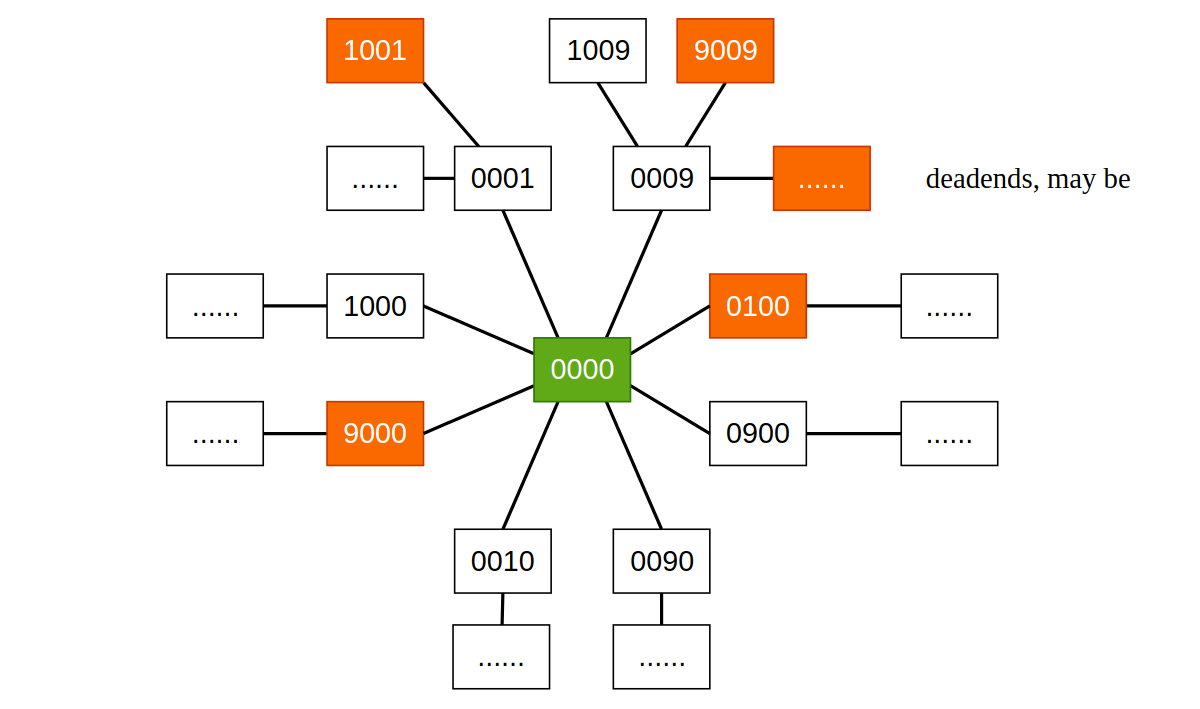https://github.com/SmartKeyerror/Snorlax/blob/master/leetcode/bfs/752-Open-the-Lock.cpp

• 装满其中一个桶: `9 * 10 + y`（装满 9 升桶），`10x + 4`（装满 4 升桶）
• 倒掉其中一个桶: `0 * 10 + y`（倒掉 9 升桶），`10x + 0`（倒掉 4 升桶）

• 将 9 升桶的水倒进 4 升桶: `10 * (9 - min(x, 4 - y)) + (y + min(x, 4 - y))`
• 将 4 升桶的水倒进 9 升桶: `10 * (x + min(y, 9 - x)) + (y - min(y, 9 - x))`

``````int bucketPuzzle() {
queue<int> levelQueue;

// 使用 hashmap 判定是否访问过, value 为操作次数
unordered_map<int, int> visited;

levelQueue.push(0);
visited = 0;

while (!levelQueue.empty()) {
int current = levelQueue.front();
levelQueue.pop();

int x = current / 10, y = current % 10;

vector<int> nextStatuses;

nextStatuses.push_back(9 * 10 + y);
nextStatuses.push_back(x * 10 + 4);

nextStatuses.push_back(0 * 10 + y);
nextStatuses.push_back(x * 10 + 0);

nextStatuses.push_back(10 * (9 - min(x, 4 - y)) + (y + min(x, 4 - y)));
nextStatuses.push_back(10 * (x + min(y, 9 - x)) + (y - min(y, 9 - x)));

for (int next: nextStatuses) {
if (!visited.count(next)) {
levelQueue.push(next);
visited[next] = visited[current] + 1;

if (next / 10 == 6 || next % 10 == 6)
return visited[next];
}
}
}
return -1;
}
``````

https://github.com/SmartKeyerror/Snorlax/blob/master/leetcode/bfs/BucketPuzzle.cpp

### 4. 小结

BFS 由于天然地存在“无权图最短路径问题求解”这一特性，被广泛地应用在经典人工智能和搜索领域，包括我们所描述的水桶问题、打开密码锁问题，以及未提及的其它经典人工智能问题，本质上就是路径的搜索。